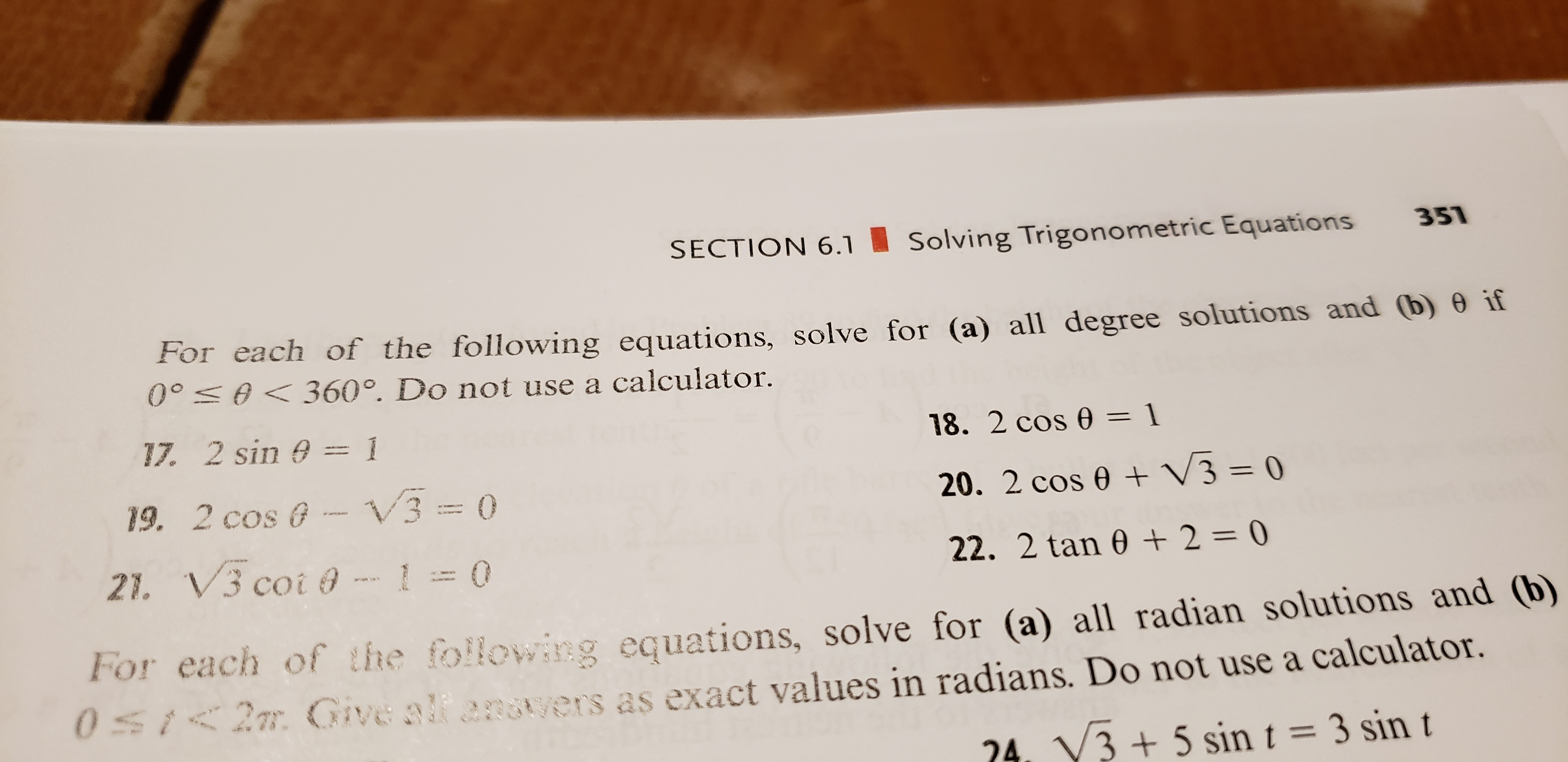# SECTION 6.1351Solving Trigonometric EquationsFor each of the following equations, solve for (a) all degree solutions and (b) 0 if00 0360°. Do not use a calculator.17. 2 sin 0118. 2 cos 0 = 1V3 = 0319. 2 cos @20. 2 cos e021. V3 coi @22. 2 tan 0 2 = 0For each of the following equations, solve for (a) all radian solutions and (b)0St2m Give alf angtyers as exact values in radians. Do not use a calculator.24. V3+ 5 sin t = 3 sin tFO

Question

2cos theda- sqrt3=0

number 19help_outlineImage TranscriptioncloseSECTION 6.1 351 Solving Trigonometric Equations For each of the following equations, solve for (a) all degree solutions and (b) 0 if 00 0360°. Do not use a calculator. 17. 2 sin 0 1 18. 2 cos 0 = 1 V3 = 0 3 19. 2 cos @ 20. 2 cos e 0 21. V3 coi @ 22. 2 tan 0 2 = 0 For each of the following equations, solve for (a) all radian solutions and (b) 0St2m Give alf angtyers as exact values in radians. Do not use a calculator. 24. V3+ 5 sin t = 3 sin t FO fullscreen
check_circleExpert Solution
Step 1

We can rewrite the equation in...

### Want to see the full answer?

See Solution

#### Want to see this answer and more?

Solutions are written by subject experts who are available 24/7. Questions are typically answered within 1 hour*

See Solution
*Response times may vary by subject and question
Tagged in

### Trigonometry